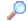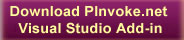SearchDirectory

Desktop Functions:

Smart Device Functions:GlossaryShow Recent ChangesSubscribe (RSS)Misc. Pages
WSASocket (ws2_32)

Summary
The WSASocket function creates a socket that is bound to a specific transport-service provider.

#### C# Signature:

[DllImport("ws2_32.dll", CharSet = CharSet.Unicode, SetLastError = true, CallingConvention = CallingConvention.StdCall)]
public static extern IntPtr WSASocket(ADDRESS_FAMILIES af, SOCKET_TYPE socket_type, PROTOCOL protocol,
IntPtr lpProtocolInfo, Int32 group, OPTION_FLAGS_PER_SOCKET dwFlags);

#### VB Signature:

Declare Function WSASocket Lib "ws2_32.dll" (TODO) As TODO

#### User-Defined Types:

{
/// <summary>
/// Unspecified [value = 0].
/// </summary>
AF_UNSPEC = 0,
/// <summary>
/// Local to host (pipes, portals) [value = 1].
/// </summary>
AF_UNIX = 1,
/// <summary>
/// Internetwork: UDP, TCP, etc [value = 2].
/// </summary>
AF_INET = 2,
/// <summary>
/// Arpanet imp addresses [value = 3].
/// </summary>
/// <summary>
/// Pup protocols: e.g. BSP [value = 4].
/// </summary>
AF_PUP = 4,
/// <summary>
/// Mit CHAOS protocols [value = 5].
/// </summary>
AF_CHAOS = 5,
/// <summary>
/// XEROX NS protocols [value = 6].
/// </summary>
AF_NS = 6,
/// <summary>
/// IPX protocols: IPX, SPX, etc [value = 6].
/// </summary>
AF_IPX = 6,
/// <summary>
/// ISO protocols [value = 7].
/// </summary>
AF_ISO = 7,
/// <summary>
/// OSI is ISO [value = 7].
/// </summary>
AF_OSI = 7,
/// <summary>
/// european computer manufacturers [value = 8].
/// </summary>
AF_ECMA = 8,
/// <summary>
/// datakit protocols [value = 9].
/// </summary>
AF_DATAKIT = 9,
/// <summary>
/// CCITT protocols, X.25 etc [value = 10].
/// </summary>
AF_CCITT = 10,
/// <summary>
/// IBM SNA [value = 11].
/// </summary>
AF_SNA = 11,
/// <summary>
/// DECnet [value = 12].
/// </summary>
AF_DECnet = 12,
/// <summary>
/// Direct data link interface [value = 13].
/// </summary>
AF_DLI = 13,
/// <summary>
/// LAT [value = 14].
/// </summary>
AF_LAT = 14,
/// <summary>
/// NSC Hyperchannel [value = 15].
/// </summary>
/// <summary>
/// AppleTalk [value = 16].
/// </summary>
AF_APPLETALK = 16,
/// <summary>
/// NetBios-style addresses [value = 17].
/// </summary>
AF_NETBIOS = 17,
/// <summary>
/// VoiceView [value = 18].
/// </summary>
AF_VOICEVIEW = 18,
/// <summary>
/// Protocols from Firefox [value = 19].
/// </summary>
AF_FIREFOX = 19,
/// <summary>
/// Somebody is using this! [value = 20].
/// </summary>
AF_UNKNOWN1 = 20,
/// <summary>
/// Banyan [value = 21].
/// </summary>
AF_BAN = 21,
/// <summary>
/// Native ATM Services [value = 22].
/// </summary>
AF_ATM = 22,
/// <summary>
/// Internetwork Version 6 [value = 23].
/// </summary>
AF_INET6 = 23,
/// <summary>
/// Microsoft Wolfpack [value = 24].
/// </summary>
AF_CLUSTER = 24,
/// <summary>
/// IEEE 1284.4 WG AF [value = 25].
/// </summary>
AF_12844 = 25,
/// <summary>
/// IrDA [value = 26].
/// </summary>
AF_IRDA = 26,
/// <summary>
/// Network Designers OSI &amp; gateway enabled protocols [value = 28].
/// </summary>
AF_NETDES = 28,
/// <summary>
/// [value = 29].
/// </summary>
AF_TCNPROCESS = 29,
/// <summary>
/// [value = 30].
/// </summary>
AF_TCNMESSAGE = 30,
/// <summary>
/// [value = 31].
/// </summary>
AF_ICLFXBM = 31
}

public enum SOCKET_TYPE : short
{
/// <summary>
/// stream socket
/// </summary>
SOCK_STREAM = 1,

/// <summary>
/// datagram socket
/// </summary>
SOCK_DGRAM = 2,

/// <summary>
/// raw-protocol interface
/// </summary>
SOCK_RAW = 3,

/// <summary>
/// reliably-delivered message
/// </summary>
SOCK_RDM = 4,

/// <summary>
/// sequenced packet stream
/// </summary>
SOCK_SEQPACKET = 5
}

public enum PROTOCOL : short
{//dummy for IP
IPPROTO_IP = 0,
//control message protocol
IPPROTO_ICMP = 1,
//internet group management protocol
IPPROTO_IGMP = 2,
//gateway^2 (deprecated)
IPPROTO_GGP = 3,
//tcp
IPPROTO_TCP = 6,
//pup
IPPROTO_PUP = 12,
//user datagram protocol
IPPROTO_UDP = 17,
//xns idp
IPPROTO_IDP = 22,
//IPv6
IPPROTO_IPV6 = 41,
//UNOFFICIAL net disk proto
IPPROTO_ND = 77,

IPPROTO_ICLFXBM = 78,
//raw IP packet
IPPROTO_RAW = 255,

IPPROTO_MAX = 256
}

public enum OPTION_FLAGS_PER_SOCKET : short
{
// turn on debugging info recording
SO_DEBUG = 0x0001,
SO_ACCEPTCONN = 0x0002,
// keep connections alive
SO_KEEPALIVE = 0x0008,
SO_DONTROUTE = 0x0010,
// permit sending of broadcast msgs
// bypass hardware when possible
SO_USELOOPBACK = 0x0040,
// linger on close if data present
SO_LINGER = 0x0080,
// leave received OOB data in line
SO_OOBINLINE = 0x0100,
SO_DONTLINGER = (int)(~SO_LINGER),

///*
// */
// send buffer size
SO_SNDBUF = 0x1001,
SO_RCVBUF = 0x1002,
// send low-water mark
SO_SNDLOWAT = 0x1003,
SO_RCVLOWAT = 0x1004,
// send timeout
SO_SNDTIMEO = 0x1005,
SO_RCVTIMEO = 0x1006,
// get error status and clear
SO_ERROR = 0x1007,
// get socket type
SO_TYPE = 0x1008,

///*
// * WinSock 2 extension -- new options
// */
// ID of a socket group
SO_GROUP_ID = 0x2001,
// the relative priority within a group
SO_GROUP_PRIORITY = 0x2002,
// maximum message size
SO_MAX_MSG_SIZE = 0x2003,
// WSAPROTOCOL_INFOA structure
SO_PROTOCOL_INFOA = 0x2004,
// WSAPROTOCOL_INFOW structure
SO_PROTOCOL_INFOW = 0x2005,
// configuration info for service provider
PVD_CONFIG = 0x3001,
// enable true conditional accept: connection is not ack-ed to the other side until conditional function returns CF_ACCEPT
SO_CONDITIONAL_ACCEPT = 0x3002
}

#### Alternative Managed API:

Do you know one? Please contribute it!

None.

#### Sample Code:

Documentation

Do you have...

• helpful tips or sample code to share for using this API in managed code?
• corrections to the existing content?
• variations of the signature you want to share?
• additional languages you want to include?

Select "Edit This Page" on the right hand toolbar and edit it! Or add new pages containing supporting types needed for this API (structures, delegates, and more).Access PInvoke.net directly from VS:Terms of UseEdit This PageFind ReferencesShow Printable VersionRevisions Show changes